# Tail recursion

Recursion is when a function calls itself. In practice, it can be clearer than a loop for expressing a process that builds on itself. The best algorithmic candidates for recursion are the ones that involve some sort of branching, since that can easily be accomplished by the function calling itself multiple times. The following code illustrates how this might be done.

```void CheckAllBranches(Node* node) //assume this is a node in a binary tree
{
if(node->hasRightChild())
{
CheckAllBranches(node->RightChild());
}

if(node->hasLeftChild())
{
CheckAllBranches(node->LeftChild());
}

//do the checking here
}```

Some people argue that recursive functions have no clarity advantages over loops, but I think that may just be a matter of preference. There are many valid criticisms of recursion, like “they suffer from call overhead” or “they can create an exception if they use all of the memory”. This last point is especially valid for deep recursive functions, one’s which call themselves a very large number of times. When a program creates a scope, like calling a function, it adds a frame to the call stack. It’s rare that the stack grows beyond the allowed limit (especially on Unix, see Addendum) but when it does, a stack overflow exception is generated and the program is terminated.

Consider the following unoptimized code where I illustrate a deep recursion.

```#include <tchar.h>
#include <iostream>

int Recurse(int count)
{
count--;
std::cout << count;
if(count == 0)
return count;

return Recurse(count);
}

int _tmain(int argc, _TCHAR* argv[])
{
Recurse(500000); //that's a pretty deep recursion!!!!!
return 0;
}```

Basically, the Recurse() function calls itself a half million times, which puts a half million frames on the stack. This is enough that we’ll get a stack overflow exception, so this code will fail at runtime.

You may have noticed that I wrote ‘unoptimized’ earlier, and that’s an important caveat since the compiler (if you ask it to) will try to optimize away the risk of deep recursion. In this case it will do something called a Tail Recursion or Tail Call optimization. The above code is written in such a way that the last statement is the recursive call, because of this, the compiler recognizes that it doesn’t need the previous stack frame to persist beyond the next recursive call. Instead of adding a new stack frame for each recursion the program could just uses one frame which is poped and then recreated with the new input to Recurse(). Consider an alternative, but functionally identical, implementation of the Recurse() method.

```int Recurse(int count)
{
count--;
std::cout << count;

if(count != 0)
count = Recurse(count);

return count;
}```

Since this implementation doesn’t have the Recurse() call as the last statement, it’s not a candidate for the tail call optimization. Since the result of Recurse() needs to be assigned to `count` after the Recurse() method returns, the current stack frame is required until all the deeper frames are resolved. A modern compiler, with optimizations turned on, will still likely optimize this function so that it doesn’t need to create a half million stack frames, perhaps by seeing that `count` doesn’t change after the assignment and doing some rearrangement so it can tail optimize.

Consider trying to observe the optimization by placing an instance of this class within the Recurse() method.

```static int stackCount = 0;
struct StackChangeNotifier
{
StackChangeNotifier()
{
stackCount++;
stackCountNumber = stackCount;
for(int i = stackCountNumber; i > 0; i--)
{
std::cout << ' ';
}

std::cout << "stack allocating, count : " << stackCountNumber << std::endl;
}

~StackChangeNotifier()
{
stackCount--;
for(int i = stackCountNumber; i > 0; i--)
{
std::cout << ' ';
}
std::cout << "stack deallocating, count : " << stackCountNumber << std::endl;
}
protected:
int stackCountNumber;
};```

If we wrote it into the Recurse() method in the following way what would you expect the output to be?

```#include <tchar.h>
#include <iostream>

int Recurse(int count)
{
StackChangeNotifier scn;
count--;
std::cout << "count: " << count << std::endl;

if(count == 0)
return count;

return Recurse(count);
}

int _tmain(int argc, _TCHAR* argv[])
{
Recurse(10); //only a few recursions
return 0;
}```

If no optimizations were performed, it would look like the following because each lower stack frame exists until the ones above it are popped.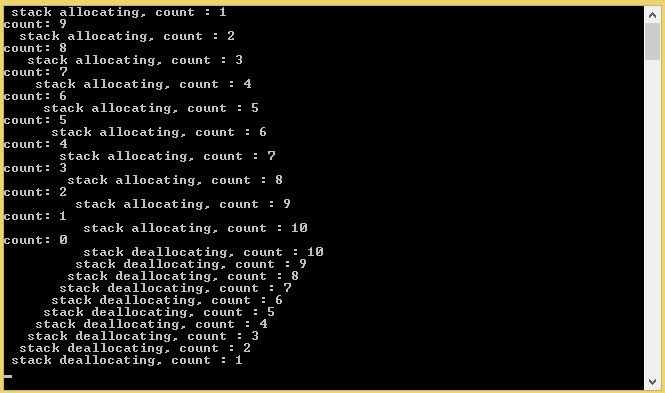In a tail optimized version it might look like the following because the result of the previous stack frame is passed into the next recursion without persisting the last frame.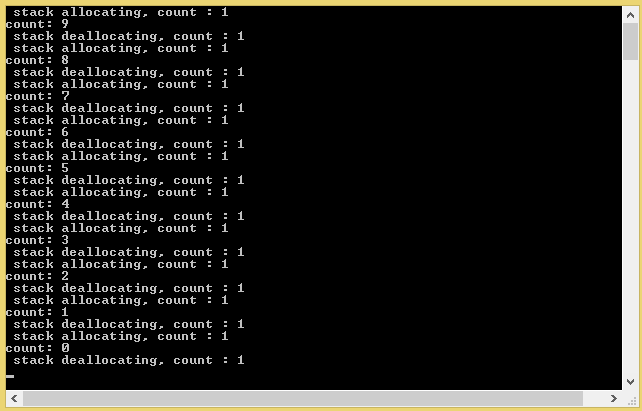It doesn’t actually look like this though because the explicit destructor of the StackChangeNotifier signals to the optimizer that it can’t do a Tail Recursion Optimization. The above program output was faked, sorry for deceiving you. The optimization will only take place if the compiler believes there are no side effects from its change, and doing anything in the destructor of a class suggests to the compiler that there is a side effect.

Lets take a look at what the compiler actually produces when it compiles the following Recurse() function.

```int Recurse(int count)
{
count--;
std::cout << count;
if(count == 2)
return count;

return Recurse(count);
}```

I’m going to show you the disassembly of this program via a tool called IDA. I’ve provided a short table of the relevant keywords in case your assembly is rusty.

 Opcode Description cmp compares two items via subtraction and sets some flags based on the result (notably the zero flag if they are the same) jnz jump to an address if the zero flag is not set jmp unconditional jump to an address sub subtract one number from another. In our code “sub eax, 1”, the eax register (just think variable) is being decremented. call executes a function

The syntax for this display is x_y,z where x is the operation, _ is a tab, y is what is being operated on, the ‘,’ is the comma, and z is whatever is being applied to y. Some operations are unary or have no operands at all.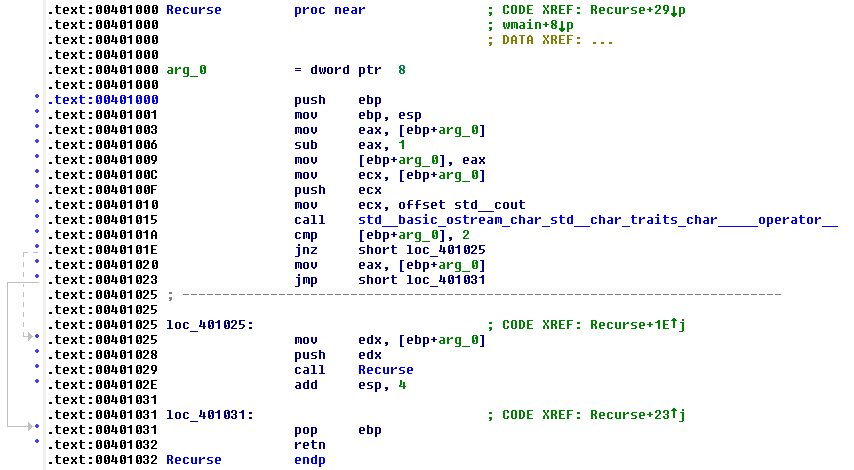The important things to notice are the looping conditions. We decrement the “count” variable (unnamed in the assembly) at offset 401006. If we have not counted down completely by line offset 40101A then we jump down to the calling code at offset 401025 and Recurse again (we only count down to 2 in this case because it made finding the `cmp` call easier for me). If we have counted down completely then the zero flag is set and the jnz is passed over and we unconditionally jump to our return under offset 401031. This is exactly what we’d expected to see in an unomptimized compilation.

There area few different options for optimization in msvc; size, speed, or full. When we optimize for size we get the following assembly. Notice the use of `dec esi` instead of `sub eax, 1` and the lack of `pop` and `push` within the range of the `jnz` operand.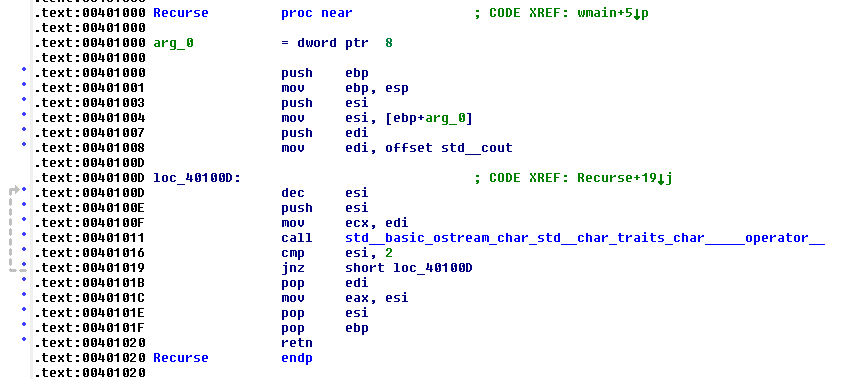You might be thinking, if there are no pop and push operations is this actually creating stack frames? No, it’s not. The compiler has done a fairly common thing where it will roll simple recursions into loops. Can all recursions be transformed into simple loops? To the best of my knowledge, they can’t but I’ll explore this in a future post.

Here’s the disassembly of the same code as before but optimized for speed.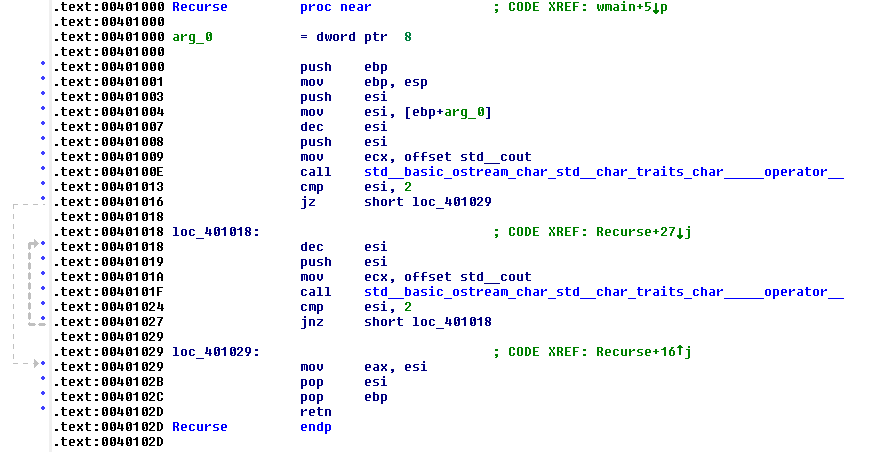This does an interesting thing in that it does an initial check for if the input is already decremented enough using the `cmp` and `jz` operations, and if not, then it falls through into the same loop we saw previously. We get the same disassembly if we use the “full” optimization option.

It seems like this function just gets converted into a loop, so how do we get “real” tail recursion from the compiler where we create a stack frame for the function, execute it, save the result, pop the frame and create another to pass the previous saved value into? If we consider that adding and removing stack frames has some cost, then we might think to just reuse the same frame over again, right? I mean, the goal is to optimize the code, so why only partially optimize when such an obvious improvement exists? The code we’ve been looking at is exactly what we’d expect if we got tail call optimization and reused the stack frame, it just happens that this looks like a loop. So we have been getting tail call optimizations, they just reuse the current frame instead of suffering the overhead of saving state and creating a new frame every time.

Thanks for reading, I hope this has been an enjoyable installment.

• As it turns out the Windows OS requires that the stack size be encoded into the executable and the default size is 1MB. Unix doesn’t encode the size in its executables, and instead each distro defines its stack size. If you’re running Unix, try typing `ulimit -s` into the terminal to see what your “user limit” is for your stacks.